How to Count the Average Between Two Dates in Excel

In our daily work, we usually have the problem of counting the average of a period. If the given dates are continuous, we can handle this situation very well, and if the dates are discontinuous, how do we count the average for different periods? In this article, we will use some examples to show you how to count the average for two dates by Average formula in different situations.

Count the Average Between Continuous Dates

First prepare a table with given dates and sales.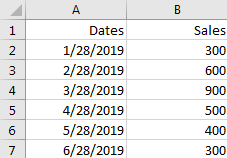You can see the given dates are continuous, so we can count average by Average formula directly.

Step 1: In B7, enter the formula =AVERAGE(B2:B4) to count the average between dates 1/28/2019 and 3/28/2019.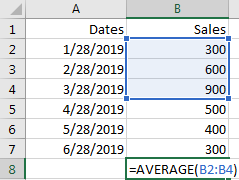Step 2: Click Enter to get the result.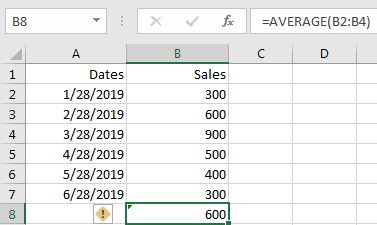Count the Average Between Discontinuous Dates

If in above table the dates are discontinuous and unordered, how can we count the average of sales? See screenshot below.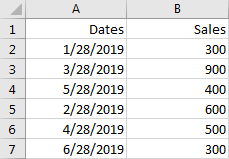You can follow below steps to count the average for two discontinuous dates.

Step 1: Prepare another table to save the Start Date, End Date and Average.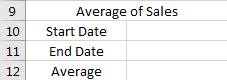Step 2: In Start Date enter the start date of the period you want to count the average. In End Date enter the end date of the period you want to count the average. For example, we count the average for period 2/28/2019 and 4/28/2019.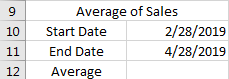Step 3: In Average, enter the formula =AVERAGE(IF((A2:A7>=B10)*(A2:A7<=B11),B2:B7)).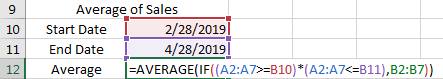In above formula, A2:A7 is the given date range, B10 is the start date, B11 is the end date, B2:B7 is the sales range.

Step 4: After entering the formula, click Ctrl + Shift + Enter to get the result.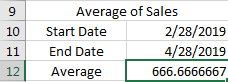Check the formula bar, you can find the formula is displayed as below. That’s an array formula.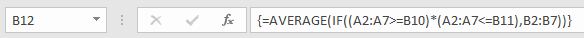Notes:

1. In step 4, if we just click Enter after entering the formula, then we will get an error here. That’s because above formula is an array formula.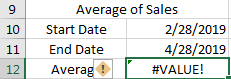2. If we just want to use a normal formula to count the average and click Enter to get the result, we can use below formula =SUMPRODUCT(–(A2:A7>=B10),–(A2:A7<=B11),B2:B7)/SUMPRODUCT(–(A2:A7>=B10),–(A2:A7<=B11)). We can get the same result.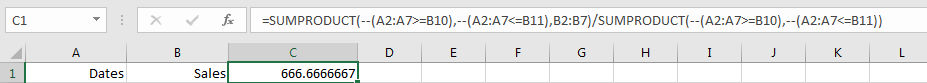Related Functions

• Excel SUMPRODUCT function
The Excel SUMPRODUCT function multiplies corresponding components in the given one or more arrays or ranges, and returns the sum of those products.The syntax of the SUMPRODUCT function is as below:= SUMPRODUCT (array1,[array2],…)…
• Excel IF function
The Excel IF function perform a logical test to return one value if the condition is TRUE and return another value if the condition is FALSE. The IF function is a build-in function in Microsoft Excel and it is categorized as a Logical Function.The syntax of the IF function is as below:= IF (condition, [true_value], [false_value])….
• Excel AVERAGE function
The Excel AVERAGE function returns the average of the numbers that you provided.The syntax of the AVERAGE function is as below:=AVERAGE (number1,[number2],…)….

Related Posts

Case Sensitive Lookup with SUMPRODUCT and EXACT

Today, we will show you how to use SUMPRODUCT and EXACT to perform a case sensitive exact match. In this article, we provide a simple example to calculate bonus for employees whose names are case-sensitive. If you meet similar scenarios ...

Average per Week by Formula in Excel

We usually apply AVERAGE function or relevant functions to return average directly in Excel worksheet. But in some situations, only applying average relevant functions cannot figure out our problem. Sometimes we can create a formula with functions and mathematical operation ...

How to Sum if Equal to Many Items or A Range in Excel

If we want to sum numbers from a range with criteria, we often select SUMIF of SUMIFS functions as the first choice to create a formula. The criteria can be a number or an array or a collection of some ...

How to Sum if Equal to X or Y in Excel

In daily work, if we want to sum numbers from a range, and only sum the numbers which being equal to X or Y in the range, we can create a formula with Excel build-in functions to get the result. ...

How To Sum the Largest N Values in Excel

Sometimes we may want to sum the largest N numbers or top N numbers in a range. In this article, we will show you the method of “SUM the Largest N Numbers” by a simple formula which consist of SUMPRODUCT ...

How to Sum for Cell Contains Formula Only in Excel

Sometimes values are created by formulas in cells. If we want to sum values which are created by formulas from a range, but some values which are hardcoded also list in the same range, how can we filter out matched ...

How to Sum the Smallest N Values in Excel

Sometimes we may want to sum the first smallest N numbers in a range in Excel. In this article, we will show you the method of “SUM the Smallest N Numbers” by a simple formula which consist of SUMPRODUCT and ...

How to Sum by SUMPRDUCT with One Specific Criteria Multiple Columns in Excel

Sometimes we may meet the case that to sum numbers based on one specific criteria. In this article, we will show you the method to resolve this problem by formula with the help of Excel SUMPRODUCT function. SUMPRODUCT can filter ...

How to Sum by SUMPRDUCT with Specific Criteria in Excel

Sometimes we may meet the case that to sum numbers based on two or more specific criteria. In this article, we will show you the method to resolve this problem by formulas with the help of Excel SUMPRODUCT function. SUMPRODUCT ...

How to Count Unique Numeric Values in a Range in Excel

This post will guide you how to count unique numeric values in the given range in Excel 2013/2016 or Excel office 365. How do I count the unique numeric values in a list of data with some duplicate values using ...

Sidebar